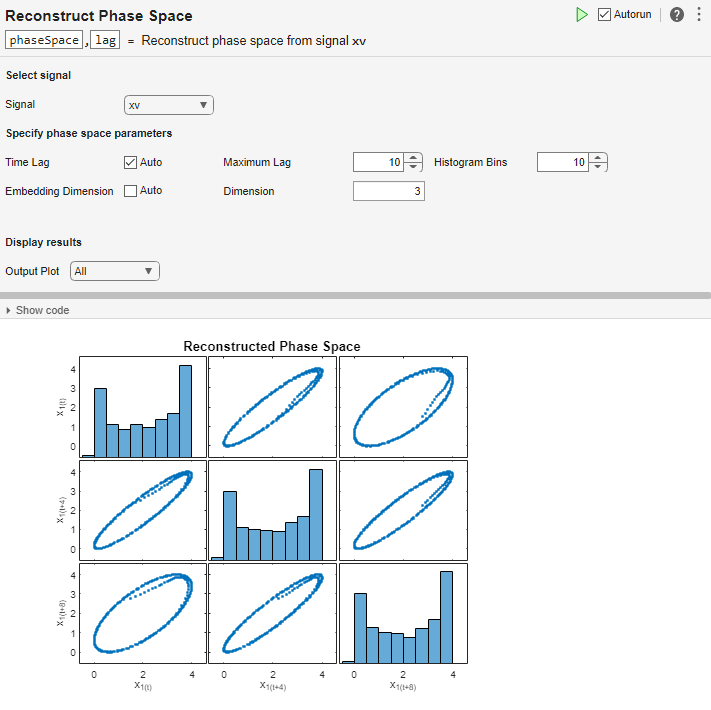Documentation

# Reconstruct Phase Space

Reconstruct phase space of a uniformly sampled signal in the Live Editor

## Description

Phase space reconstruction is useful to verify the system order and reconstruct all dynamic system variables, while preserving system properties. Reconstructing the phase space is performed when limited data is available, or when the phase space dimension and lag values are unknown. Also, the nonlinear features `approximateEntropy`, `correlationDimension`, and `lyapunovExponent` use phase space reconstruction as the first step of the computation. For more information about phase space reconstruction, see `phaseSpaceReconstruction`.To add the Reconstruct Phase Space task to a live script in the MATLAB Editor:

• On the Live Editor tab, select Task > Reconstruct Phase Space.

• In a code block in your script, type a relevant keyword, such as `phase` or `phase space`. Select `Reconstruct Phase Space` from the suggested command completions.

## Examples

expand all

Use the Reconstruct Phase Space task in the Live Editor to interactively reconstruct the phase space of a uniformly sampled signal. Experiment with different values for lag, embedding dimension, histogram bins and distance threshold. The task automatically generates code reflecting your selections. Open this example to see a preconfigured script containing the Reconstruct Phase Space task.

For this example, consider '`uavPositionData.mat`' which contains signal xv which is the x-component of a 3-D path traversed by an unmanned aerial vehicle (UAV). The x, y, and z coordinates define a circle of 2-m radius at 0.75-m altitude.

`load('uavPositionData.mat','xv')`

To reconstruct the phase space of the signal `xv`, open the Reconstruct Phase Space task in the Live Editor. On the Live Editor tab, select Task > Reconstruct Phase Space. In the task, select signal `xv`.Clear the Time Lag check box if you want to use your own values in the Maximum Lag and Histogram Bins fields. For this example, leave the box checked to calculate the lag using Average Mutual Information (AMI). Since dimension is known, clear the Embedding Dimension field and specify dimension as 3.Evaluate whether the reconstructed phase space preserves the system dynamics with the assigned values by observing the output plots. You can toggle between the display type by choosing between `Individual` or `All` in the Output Plot dropdown menu.The task generates code in your live script. The generated code reflects the parameters and options you select, and includes code to generate the type of plot you specify. To see the generated code, clickat the bottom of the task parameter area. The task expands to display the generated code.By default, the generated code uses `phaseSpace` as the name of the output variable. To specify a different output variable name, enter a new name in the summary line at the top of the task. For instance, change the name to `pSpace`.The task updates the generated code to reflect the new variable name, and the new variable `pSpace` appears in the MATLAB workspace. You can use the reconstructed phase space to identify condition indicators like Lyapunov exponent or correlation dimension.

## Parameters

#### Select Signal

Select a uniformly sampled time-domain signal in array or timetable format.

#### Specify Phase Space Parameters

Check to use Average Mutual Information (AMI) algorithm to compute time lag. Clear to try your own value of Maximum Lag and Histogram Bins. If the time delay is too small, random noise is introduced in the states. In contrast, if the lag is too large, the reconstructed dynamics do not represent the true dynamics of the time series.

Maximum value of lag used to estimate the time delay using the Average Mutual Information (AMI) algorithm.

Number of bins for discretization to compute lag using the AMI algorithm. Set the value of Histogram Bins based on the length of your signal.

Check to use Percent False Neighbors (PFN) algorithm to automatically compute embedding dimension.

Maximum value of embedding dimension used in the dimension estimation with Percent False Neighbors (PFN) algorithm.

Distance ratio threshold for determining two points as false neighbors using Percent False Neighbors (PFN) algorithm. For more information, see `phaseSpaceReconstruction`.

Percent false neighbors threshold for detecting embedding dimension using PFN algorithm. To specify percent false neighbors, check the Embedding Dimension check box. For more information, see `phaseSpaceReconstruction`.

#### Visualize Results

Number of output plots to display. To toggle between the reconstructed plot and the histogram plot, and to go through each plot, select `Individual`. To display both plots in the Live Editor, select `All`. To hide plots, select `None`.